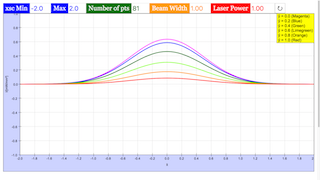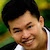Intro Page

Laser Beam Profile

Developed by E. Behringer

This set of exercises guides the student to model the results of an experiment to determine the profile of a laser beam using a knife-edge technique. It requires the development of the model of the knife-edge profile, and fitting of the model profile to experimental data. Here, the computational tasks are handled by built-in functions of the computational tool being used to complete these exercises.

 Subject Area Waves & Optics Beyond the First Year Python Students who complete this set of exercises will be able to express an equation predicting the profile of a laser oscillating in the TEM00 mode in terms of dimensionless (“scaled”) variables suitable for coding (Exercise 1); produce both line plots and contour plots of the (scaled) irradiance of the beam versus (scaled) position(s) (Exercises 1 and 2); develop a model of, and plot, the knife-edge profile of the laser beam (Exercise 3); and fit the model, i.e., of the irradiance versus knife-edge position, to experimental data (Exercise 4) 120 min
Exercise 1

### EXERCISE 1: THE TEM00MODE: “LINE CUTS”

The irradiance  (power per unit area) of a laser oscillating in the TEM mode can be expressed as

where  is the maximum irradiance of the beam and  is a parameter that describes the width (i.e., the spatial extent) of the beam. You can show that when , the locations  at which  are . Show that when  and , the irradiance is  and show that the above expression for the irradance is equivalent to

where the “scaled variables”  and  are defined as . We can relate this expression to the total power  transmitted by the beam:

(a) Assume  mW and  mm to generate a line plot of the irradiance  on the vertical axis versus  on the horizontal axis for  for different values of  and .

(b) Repeat (a) but now plot the irradiance divided by the maximum irradiance for that particular value of  (in other words, plot ) versus  on the horizontal axis. Comment on the resulting plot.

Beam_Profile_Exercise_1.py

#

# Beam_Profile_Exercise_1.py

#

# This file will generate a plot of

# the irradiance I(x,y) of the TEM00 Gaussian Mode of a laser

# as a function of scaled x for several different values of scaled y.

#

# This file will also generate a plot of

# the scaled irradiance I(x,y) of the TEM00 Gaussian Mode of a laser

# as a function of scaled x for several different values of scaled y.

#

# Written by:

#

# Ernest R. Behringer

# Department of Physics and Astronomy

# Eastern Michigan University

# Ypsilanti, MI 48197

# (734) 487-8799

# This email address is being protected from spambots. You need JavaScript enabled to view it.

#

# 20160628 ERB

#

from pylab import xlim,xlabel,ylim,ylabel,show,plot,figure,grid,legend

from numpy import linspace,exp,pi,zeros

# Generate scaled x and y values

max_xsc = 2.0 # maximum value of x position [mm]

min_xsc = -2.0 # minimum value of x position [mm]

Npts = 81

xsc = linspace(min_xsc,max_xsc,Npts) # array of scaled x values

ysc = [0.0,0.2,0.4,0.6,0.8,1.0]

# other inputs

w0 = 1.00 # beam width [mm]

P_0 = 1.00 # laser power [mW]

I_0 = 2.00*P_0/(pi*w0*w0) # maximum irradiance [mW/mm2]

# Set up array of irradiances

I = zeros((6,Npts))

for i in range(0,6):

I[i] = I_0 * exp(-2.0*(xsc*xsc + ysc[i]*ysc[i]))

# Generate the line plot of irradiance versus scaled x values

# for different scaled y values

figure()

# Here we plot unscaled I versus the scaled x for different scaled y values,

plot(xsc,I,'mo-',label='$$\\tilde y = 0.0$$',markevery=8)

plot(xsc,I,'bD-',label='$$\\tilde y = 0.2$$',markevery=4)

plot(xsc,I,'g>-',label='$$\\tilde y = 0.4$$',markevery=2)

plot(xsc,I,'ys-',label='$$\\tilde y = 0.6$$',markevery=5)

plot(xsc,I,color='#ffaa00',marker='^',linestyle='-',label='$$\\tilde y = 0.8$$',markevery=4)

plot(xsc,I,'r*-',label='$$\\tilde y = 1.0$$',markevery=3)

# Define the limits of the horizontal axis

xlim(min(xsc),max(xsc))

# Label the horizontal axis, with units

xlabel("$$\\tilde x$$", size = 16)

# Define the limits of the vertical axis

ylim(min(I),max(I))

# Label the vertical axis, with units

ylabel("$$I$$ [mW/mm$$^2$$]", size = 16)

grid('on')

legend()

show()

# Generate the line plot of scaled I versus scaled x

figure()

# Here we plot scaled I versus scaled x for different scaled y values,

plot(xsc,I/max(I),'mo-',label='$$\\tilde y = 0.0$$',markevery=8)

plot(xsc,I/max(I),'bD-',label='$$\\tilde y = 0.2$$',markevery=4)

plot(xsc,I/max(I),'g>-',label='$$\\tilde y = 0.4$$',markevery=2)

plot(xsc,I/max(I),'ys-',label='$$\\tilde y = 0.6$$',markevery=5)

plot(xsc,I/max(I),color='#ffaa00',marker='^',linestyle='-',label='$$\\tilde y = 0.8$$',markevery=4)

plot(xsc,I/max(I),'r*-',label='$$\\tilde y = 1.0$$',markevery=3)

# Define the limits of the horizontal axis

xlim(min(xsc),max(xsc))

# Label the horizontal axis, with units

xlabel("$$\\tilde x$$", size = 16)

# Define the limits of the vertical axis

ylim(0.0,1.0)

# Label the vertical axis, with units

ylabel("$$I/I_{max,\\tilde{y}}$$", size = 16)

grid('on')

legend()

show()

### Translations

Code Language Translator Run### Software Requirements

SoftwareRequirements

 Android iOS Windows MacOS with best with Chrome Chrome Chrome Chrome support full-screen? Yes. Chrome/Opera No. Firefox/ Samsung Internet Not yet Yes Yes cannot work on some mobile browser that don't understand JavaScript such as..... cannot work on Internet Explorer 9 and below

### CreditsFremont Teng; Loo Kang Wee

[text]

[text]

Research

[text]

[text]

[text]